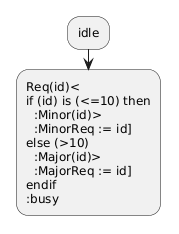# Support of any shape in any diagram

asked Jan 15, 2014

Hello,

The UML notation is quite rich in that it allows a relatively wide variety of shapes in some diagrams.

For instance, SDL-like signal reception and emission are allowed in activity diagrams (supported in PlantUML activity2/beta), but also in state diagrams (see figures 15.44 page 587 and 15.45 page 588 of UML 2.4.1 specification).

Directly supporting all UML presentation options in PlantUML is probably quite complex.

However, it may be possible to simply allow any diagram to use any (supported) shape (e.g., using skinparams or stereotypes or a combination thereof).

For instance, figure 15.44 mentionned above could be specified in the following way:

@startuml

state "Req(Id)" as ReqId <<sdlreceive>>
state "Minor(Id)" as MinorId <<sdlsend>>
state "MinorReq := Id;" as MinorReq <<rect>>
state "Major(Id)" as MajorId <<sdlsend>>
state "MajorReq := Id;" as MajorReq <<rect>>

state j <<junction>>
state c <<choice>>

Idle --> ReqId
ReqId --> c
c --> MinorId : [Id <= 10]
MinorId --> MinorReq
c --> MajorId : [Id > 10]
MajorId --> MajorReq
MinorReq --> j
MajorReq --> j
j --> Busy
@enduml

Note that some nodes (such as ReqId, MinorId, and MinorReq) are declared as "states", although they are not states. It may be interesting to introduce a generic "node" keyword for such cases (i.e., arbitrary nodes with user-selected shapes), but this is not absolutely necessary.

Also, note the use of <<junction>>, which is currently not supported by PlantUML. Therefore, <<backcircle>> may be more appropriate as generic shape identifier.

Thanks,

Fred

answered Jan 15, 2014 by (279,680 points)

For some reason, we try to promote the syntax activity2/beta over legacy state and activity syntax. This is because we find this syntax easier to use than the legacy one.

So you can/should use the new activity2/beta syntax for both activity and state diagrams (don't know if it's ok for you).

@startuml
:idle;
:Req(id)<
if (id) is (<=10) then
:Minor(id)>
:MinorReq := id]
else (>10)
:Major(id)>
:MajorReq := id]
endif
:busy;
@endumlWhat do you think about it ?

commented Jan 15, 2014 by (540 points)
For the example I gave, I agree that activity2/beta is indeed satisfying.
The only small issue in the example is that I would like a black circle instead of the bottom diamond.

However, in general, I believe it is still useful to support the specification of any shape for any node in any diagram. Assuming the implementation is not very complex (which may be a wrong assumption), this would solve many problems with a single technical solution.

For instance, considering "endif" as a node (the bottom diamond), one could specify its shape in the following way:

@startuml
:idle;
:Req(id)<
if (id) is (<=10) then
:Minor(id)>
:MinorReq := id]
else (>10)
:Major(id)>
:MajorReq := id]
endif (<<blackcircle>>)
:busy;
@enduml

Of course, this example is a bit extreme, because it relies on two currently unsupported features (AFAIK):
- stereotypes in activity2/beta
- the possibility to specify contents of "endif" diamond

But then, we could go back to using a state diagram (with <<sdlreceive>>, <<sdlsend>>, etc.) in which stereotypes are already available to select some shapes (e.g., <<choice>>, <<fork>>).

Another example is the use of junctions in other kinds of state diagrams:
@startuml
'state j <<junction>>
'or:
state j <<blackcircle>>
A --> j : evt
j --> B : / doSomething
j --> C : / doSomethingElse
@enduml
One way to solve this problem is to implement <<junction>> in the same way <<choice>> is currently implemented.
Another way would be to rely on a generic shape selection mechanism.

Here is another example (actually, the problem I currently have):
Using both an <<sdlreceive>> shape and a composite state in a given state diagram.
It seems that activity2/beta does not support composite nodes.
Therefore, the only possibility is to use a state diagram.

For instance, here is a simplified diagram (useless in itself) showing this possibility:
@startuml